#### Implied Variance Functions

While link functions are not unique for each distribution (see Table 41.13 for the default link functions), the distribution does determine the variance function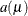. This function expresses the variance of an observation as a function of the mean, apart from weights, frequencies, and additional scale parameters. The implied variance functionsof the GLIMMIX procedure are shown in Table 41.20 for the supported distributions. For the binomial distribution, n denotes the number of trials in the events/trials syntax. For the negative binomial distribution, k denotes the scale parameter. The multiplicative scale parameteris not included for the other distributions. The last column of the table indicates whetherhas a value equal to 1.0 for the particular distribution.

Table 41.20: Variance Functions in PROC GLIMMIX

Variance function

DIST=

Distribution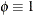BETA

beta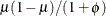No

BINARY

binary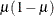Yes

BINOMIAL | BIN | B

binomial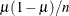Yes

EXPONENTIAL | EXPO

exponential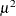Yes

GAMMA | GAM

gammaNo

GAUSSIAN | G | NORMAL | N

normal

1

No

GEOMETRIC | GEOM

geometric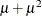Yes

INVGAUSS | IGAUSSIAN | IG

inverse Gaussian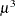No

LOGNORMAL | LOGN

lognormal

1

No

NEGBINOMIAL | NEGBIN | NB

negative binomial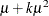Yes

POISSON | POI | P

PoissonYes

TCENTRAL | TDIST | T

t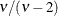No

To change the variance function, you can use SAS programming statements and the predefined automatic variables, as outlined in the following section. Your definition of a variance function will override the DIST= option and its implied variance function. This has the following implication for parameter estimation with the GLIMMIX procedure. When a user-defined link is available, the distribution of the data is determined from the DIST= option, or the respective default for the type of response. In a GLM, for example, this enables maximum likelihood estimation. If a user-defined variance function is provided, the DIST= option is not honored and the distribution of the data is assumed unknown. In a GLM framework, only quasi-likelihood estimation is then available to estimate the model parameters.